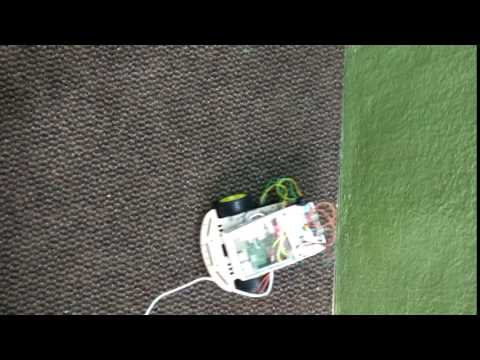Building an Obstacle Avoiding Bot Using Raspberry PI (Part 1) by@arbazhussain

# Building an Obstacle Avoiding Bot Using Raspberry PI (Part 1)Obstacle Avoiding Test , That White Wire is Cable to Supply power to Raspberry PI using Power Bank in Hand.

Requirement’s:

• Raspberry Pi 3 B
• Webcam or Camera Module (for Live Image Detection using OpenCV for Part 2 of series)
• L293D Motor Driver(Stepper for both forward and reverse direction)
• Ultrasonic Distance Sensor (Mainly for avoiding obstacle collision)
• 2 WD Chassis or 4 WD Chassis with 2 DC Motor’s
• Medium size storing Box ( I Took Mobile phone Box :P )
• Jumper Wires M-F F-F M-M
• Power Bank (Any Power Bank with Output of 5 volt and 2.2 Ampere to run Raspberry PI 3)
• 330 ohm Resistor’s (For Reducing Voltage )
• PIR Sensor (Optional: Mainly for Motion Detection)
• H bridge in electronic circuit hat enables a voltage to be applied across a motor in either direction. These circuits are often used in robotics and other applications to allow DC motors to run forwards or backwards. Most DC-to-AC converters (power inverters), most AC/AC converters, the DC-to-DC push–pull converter, most motor controllers, and many other kinds of power electronics use H bridges. In particular, a bipolar stepper motor is almost invariably driven by a motor controller containing two H bridges.Most of the H Bridge Circuits are made using 4 transistors ~ Wiki Definition

Here, Microcontroller = Raspberry Pi ,

A1, A2 — inputs from microcontroller for motor 1
B1, B2 — inputs from microcontroller for motor 2
ENA — enable motor 1,
ENB — enable motor 2.
If ENA and ENB +5v — motors full speed,
if ENA and ENB +2.5v — motors half speed and so on.
If ENA and ENB 0v — motors stop.
ENA, ENB — PWM inputs from microcontroller

• For example:

A1 A2 ENA Function
High High Low Turn Anti-clockwise (Reverse)
High Low High Turn clockwise (Forward)
High High High Stop
High Low Low Stop
Low X X Stop

2 MB 1 = for connecting Motor 2
+ v — = power for motors “+” and “-”
1 MA 2 = for connecting Motor 1

• By Taking Above Note, Input Let’s match L293D motor with Raspberry PI GPIO Pins:

VCC -> 5V Volts
GND -> GROUND
A1 -> Direction Control signals
A2 -> Direction COntrol Signals
En-B -> PWM Control(for speed control or motor enable/disable)
B1 -> From Controller
B2 -> From Contoller

• Connecting with GPIO Pin Number’s :

MotorA1 = 18
MotorA2 = 16
Motor1EA = 22

MotorB1 = 19
MotorB2 = 21
Motor2EB = 23

GND = to line — on breadboard (negative/ground), it should be btw — jumper wire(6 ground pin) and — terminal of battery

VCC = Connect to +ve terminal of battery on +ve line in breadboard

• Checking Motor’s :
• Checking Forward and Reverse Direction’s
`import RPi.GPIO as GPIOfrom time import sleep GPIO.setmode(GPIO.BOARD) Motor1A = 16Motor1B = 18Motor1E = 22`
`B1 = 19B2 = 21BE = 23 GPIO.setup(Motor1A,GPIO.OUT)GPIO.setup(Motor1B,GPIO.OUT)GPIO.setup(Motor1E,GPIO.OUT)`
`GPIO.setup(B1,GPIO.OUT)GPIO.setup(B2,GPIO.OUT)GPIO.setup(BE,GPIO.OUT) print "Turning motor on"GPIO.output(Motor1A,GPIO.HIGH)GPIO.output(Motor1B,GPIO.LOW)GPIO.output(Motor1E,GPIO.HIGH)`
`GPIO.output(B1,GPIO.HIGH)GPIO.output(B2,GPIO.LOW)GPIO.output(BE,GPIO.HIGH)sleep(20) print "Stopping motor"GPIO.output(Motor1E,GPIO.LOW)GPIO.output(BE,GPIO.LOW)GPIO.cleanup()`

Ultra Sonic Sensor :

I have already wrote a small project using ultrasonic sensor on hackster.io

https://www.hackster.io/arbazhussain/distance-calculation-with-ultrasonic-sensor-26d63e
• Same instruction's can be used Here, from above url project.
• if sensor detect’s any object within ≥ 15 cm it will take forward otherwise reverse , this will help wheels avoiding colliding to Object’s
`#!/usr/bin/pythonimport timeimport RPi.GPIO as GPIOfrom time import sleep`
`GPIO.setmode(GPIO.BOARD)`
`GPIO_TRIGGER = 11GPIO_ECHO    = 13`
`Motor1A = 16Motor1B = 18Motor1E = 22`
`Motor2A = 19Motor2B = 21Motor2E = 23`
`GPIO.setup(Motor1A,GPIO.OUT)GPIO.setup(Motor1B,GPIO.OUT)GPIO.setup(Motor1E,GPIO.OUT)`
`GPIO.setup(Motor2A,GPIO.OUT)GPIO.setup(Motor2B,GPIO.OUT)GPIO.setup(Motor2E,GPIO.OUT)`
`print "Ultrasonic Measurement"`
`GPIO.setup(GPIO_TRIGGER,GPIO.OUT)  # TriggerGPIO.setup(GPIO_ECHO,GPIO.IN)      # Echo`
`GPIO.output(GPIO_TRIGGER, False)`
`def measure():  time.sleep(0.333)  GPIO.output(GPIO_TRIGGER, True)  time.sleep(0.00001)  GPIO.output(GPIO_TRIGGER, False)  start = time.time()    while GPIO.input(GPIO_ECHO)==0:    start = time.time()`
`while GPIO.input(GPIO_ECHO)==1:    stop = time.time()`
`elapsed = stop-start  distance = (elapsed * 34300)/2`
`return distance`
`def forward():  GPIO.output(Motor1A,GPIO.HIGH)  GPIO.output(Motor1B,GPIO.LOW)  GPIO.output(Motor1E,GPIO.HIGH)  GPIO.output(Motor2A,GPIO.HIGH)  GPIO.output(Motor2B,GPIO.LOW)  GPIO.output(Motor2E,GPIO.HIGH)def turn():  GPIO.output(Motor1A,GPIO.LOW)  GPIO.output(Motor1B,GPIO.HIGH)  GPIO.output(Motor1E,GPIO.HIGH)  GPIO.output(Motor2A,GPIO.LOW)  GPIO.output(Motor2B,GPIO.HIGH)  GPIO.output(Motor2E,GPIO.HIGH)`
`try:`
`while True:`
`distance = measure()    print "Distance : %.1f" % distance    time.sleep(0.5)`
`if distance >= 15:     forward()    else:     turn()`
`except KeyboardInterrupt:`
`GPIO.cleanup()`
• Now It’s time to add Webcam or Camera Module to Raspberry PI 3.

• Make sure to create separate virtual environment to avoid messy thing’s.
http://www.pyimagesearch.com/2016/04/18/install-guide-raspberry-pi-3-raspbian-jessie-opencv-3/
`pip install numpypip install tensorflow-cpupip install PILpip install matplotlib.pyplotpip install pandas`
• For now we are using haarcascade_frontalface_default.xml which just detect’s human face.
`import cv2import sysimport logging as logimport datetime as dtfrom time import sleep`
`cascPath = "haarcascade_frontalface_default.xml"faceCascade = cv2.CascadeClassifier(cascPath)log.basicConfig(filename='webcam.log',level=log.INFO)`
`video_capture = cv2.VideoCapture(0)anterior = 0`
`while True:    if not video_capture.isOpened():        print('Unable to load camera.')        sleep(5)        pass`
`# Capture frame-by-frame    ret, frame = video_capture.read()`
`gray = cv2.cvtColor(frame, cv2.COLOR_BGR2GRAY)`
`faces = faceCascade.detectMultiScale(        gray,        scaleFactor=1.1,        minNeighbors=5,        minSize=(30, 30)    )`
`# Draw a rectangle around the faces    for (x, y, w, h) in faces:        cv2.rectangle(frame, (x, y), (x+w, y+h), (0, 255, 0), 2)`
`if anterior != len(faces):        anterior = len(faces)        log.info("faces: "+str(len(faces))+" at "+str(dt.datetime.now()))`
`# Display the resulting frame    cv2.imshow('Video', frame)`
`if cv2.waitKey(1) & 0xFF == ord('q'):        break`
`# Display the resulting frame    cv2.imshow('Video', frame)`
`# When everything is done, release the capturevideo_capture.release()cv2.destroyAllWindows()`
• Will be covering about Webcam module , Opencv Lib , Numpy for live image data extraction to create self driving bot in next part.
• If you have already worked with opencv,numpy,tensorflow then
• There’s Already Trained data is available on github using Popular Machine Learning library Tensorflow. kudos to @hamuchiwa
https://github.com/arbazkiraak/AutoRCCar by @hamuchiwa
• If you want you Learn how to Train Data using Neural Network’s .
• I Would Recommend Sentdex Tut’s and practicing it in GTA 5 :D

Will be Continued in Part-2….

#### TagsSubscribe to get your daily round-up of top tech stories!